# Set Formulas for a Cell in VBA

Iqra Hasnain May 13, 2022 VBA VBA Cell

We will introduce different cell functions that can be performed in VBA to achieve different tasks with examples.

## Set Formulas for a Cell in VBA

Worksheets of excel contain cells for data storage purposes. These cells are arranged to form rows and columns in the worksheet.

While we involve cell(s) from an excel worksheet for VBA coding, we use a range that includes one or more cells. Some of the essential features related to VBA cells are the following:

• Cell range
• Writing in cells
• Intersecting cells
• Non-Contiguous cells
• Offsetting from cells
• Referring to a cell address
• Including all cells
• Changing the properties of cells
• Finding the last cell
• Finding the last row number used in a column
• Finding the last column number used in a row
• Copy-pasting a cell

## Address of a Cell in VBA

We can identify the address of a cell through A1 notation, in which we include the name of the column letter and write the row number of the cell. In VBA, the range object can be included.

Let us refer to cell `B4`, as shown below.

``````# vba
MsgBox Range("B4")
``````

Output: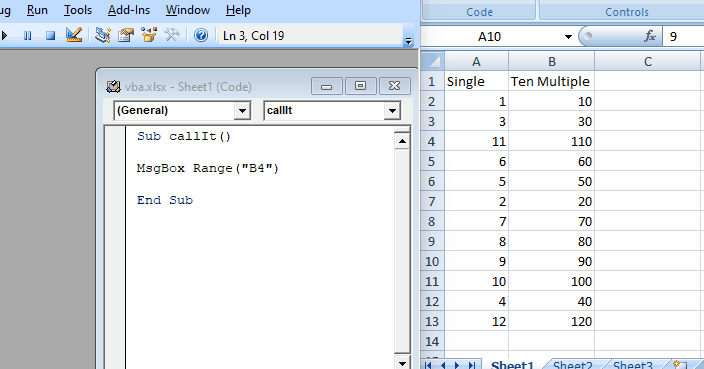As shown below, if we want to refer to a cell from another worksheet, we can easily call it by calling the sheet and getting the cell.

``````# vba
MsgBox Worksheets("Sheet2").Range("B4")
``````

Output: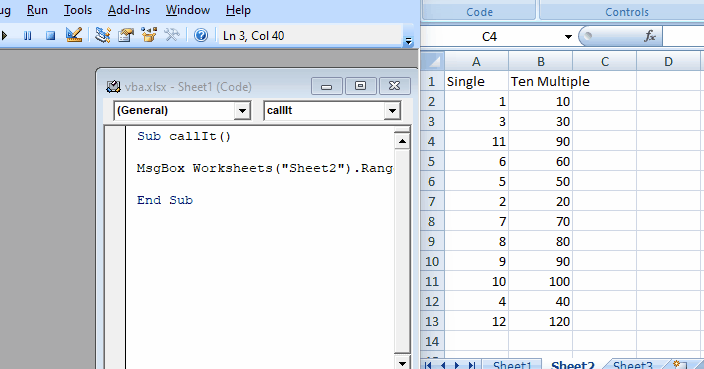We can even open another workbook and choose the worksheet from that workbook to get the value of the desired cell, as shown below.

``````# vba
MsgBox Workbooks("My WorkBook").Worksheets("Sheet2").Range("B2")
``````

Another way to write a cell address is to use the letter `R` and the row number. Next, write the letter `C` and the cell’s column number.

This method is called `R1C1 notation`. Let’s use this notation in our example, as shown below.

``````#vba
Sub callIt()

End Sub
``````

Output: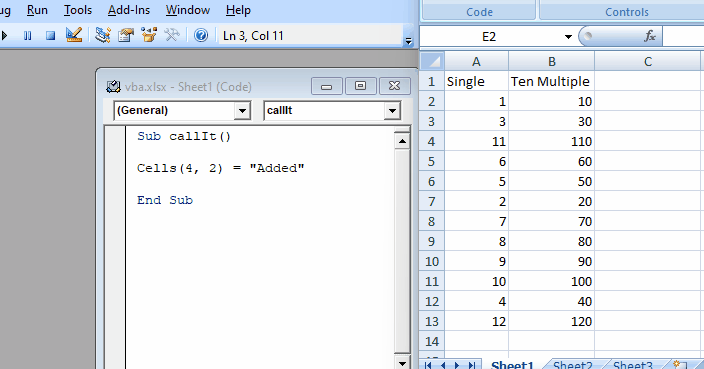## Select Cell Range in VBA

We can also select a range of cells by giving range values to the function `Range` below.

``````# vba
Range("A1:B13")
``````

We can use the above function to change the style of cells present in that range. Let us go through an example in which we will change the border color of the cells from that range, as shown below.

``````# vba
Sub callIt()

Range("A1:B13").Borders.Color = RGB(255, 0, 255)

End Sub
``````

Output: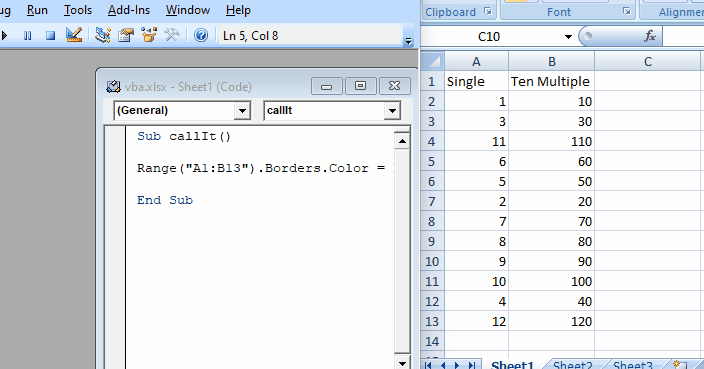As you can see from the above example, we can easily change the styles using the range function by providing the values of cells.

## Write in Cells in VBA

There are many scenarios where we may want to edit or add value to a particular cell. Using VBA, replacing the cell value or adding the cell value is very easy; we can use the `range()` function.

Then, let us use the `range()` function to add a value and change it.

``````# vba
Sub callIt()

End Sub
``````

Once the value is added, we will change the value. And we will try to replace the same cell as shown below.

``````# vba
Sub callIt()

Range("B4") = "Value Changed"

End Sub
``````

Output: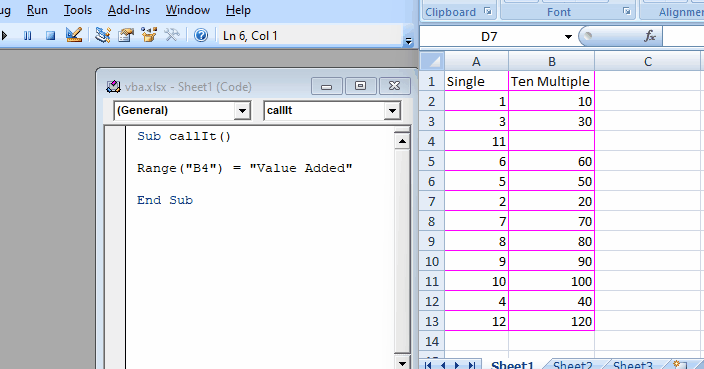We can also use the `Cells` method to perform the same function.

``````# vba
Sub callIt()

Cells(4, 2) = "Value"

End Sub
``````

Output: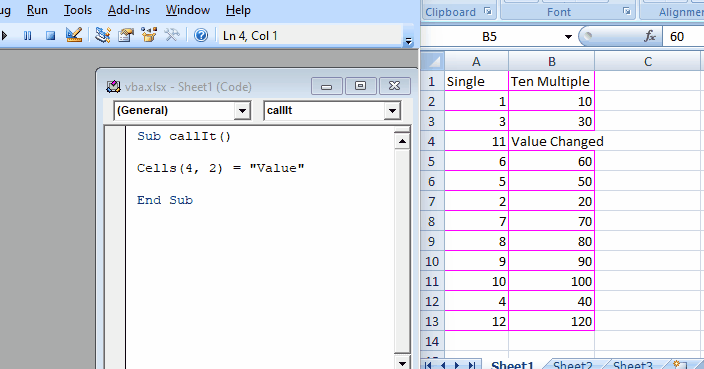If we want to store a range of values, using an array is better than using a simple variable.

## Read From Cells in VBA

If we want to read values from a cell, we can use 2 functions. One is the `Range`, and the other is the `Cells`. By calling the cell’s address, we can get its value.

We can use the `MsgBox` method to display our value. Let us go through an example and fetch the value of `B4` using the `Cells` method first.

``````# vba
Sub callIt()

cellValue = Cells(4, 2)

MsgBox (cellValue)

End Sub
``````

Output: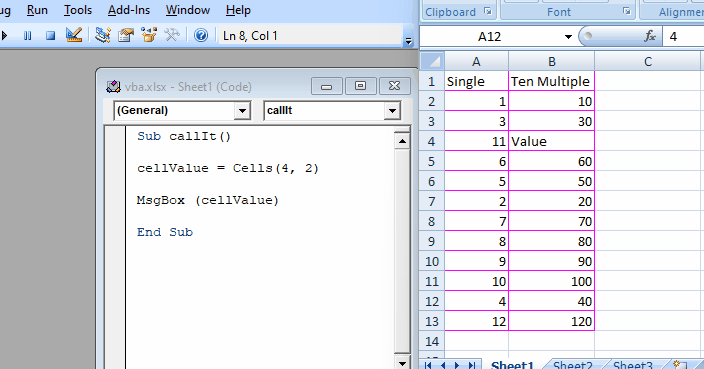Let us try to achieve the same thing using the `range()` method below.

``````# vba
Sub callIt()

cellValue = Range("B4")

MsgBox (cellValue)

End Sub
``````

Output: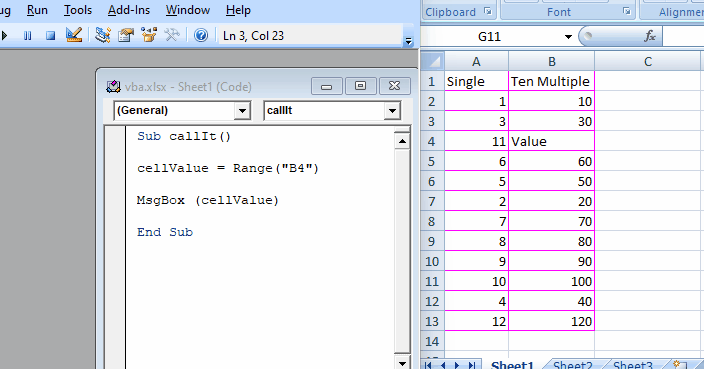As you can see from the above example, it is very easy to read the values of cells using these two functions.

## Intersect Cells in VBA

While working in VBA to create formulas for excel sheets, we may need to find the intersection of 2 ranges and perform specific tasks there. Or we want to place a particular value at the intersection of these 2 ranges.

Space is used between cell addresses and the VBA for the intersecting process.

As shown below, we will go through an example in which we will add a value in the range cells, common between both ranges.

``````# vba
Sub callIt()

Range("A1:B10 B1:C10") = "Common Cells"

End Sub
``````

Output: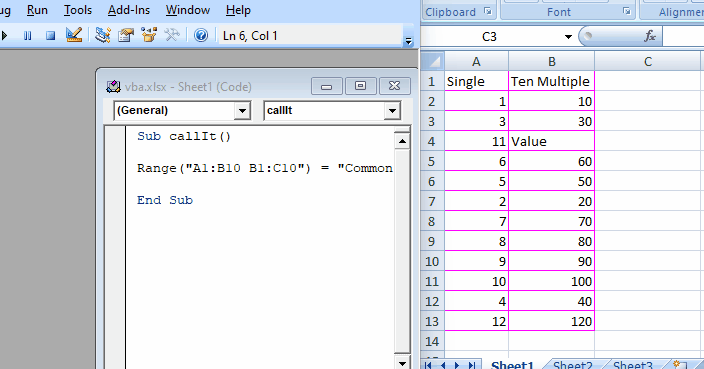As you can see from the above example, we can easily add or replace the values between the cells that intersect between two ranges.

## Replace Values of Non-Contiguous Cells in VBA

There may be some situations where we have to replace the values of the non-contiguous cells. We can easily do that using the range for multiple cells or a single cell.

For non-contiguous cells, put a comma (`,`) between different cell addresses. Let us go through an example in which we will replace the values of specific cells, as shown below.

``````# vba
Sub callIt()

Range("A2,C3,B5") = 10

End Sub
``````

Output: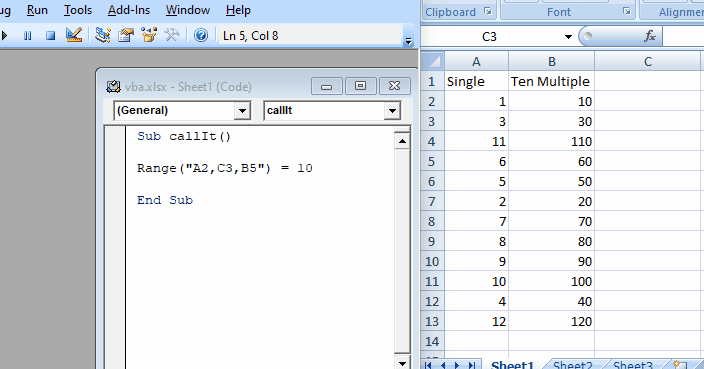Now, let us change the value of a single non-contiguous cell, as shown below.

``````# vba
Sub callIt()

Cells(5, 2) = "Hello"

End Sub
``````

Output: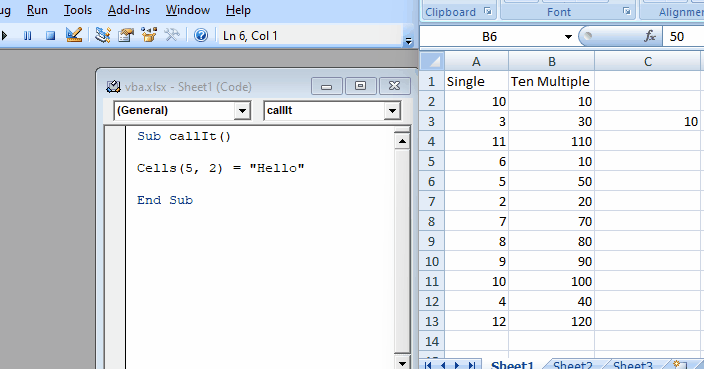## Offset From a Cell in VBA

There may be a situation while working on VBA with excel sheets where we may have a reference cell from which we want to get some offset cell value or replace the value of the cell based on the one cell address.

We can use the `offset` method to achieve this scenario smoothly.

Let us go through an example in which we will use this function to move 1 column and 1 row using the `range` method as shown below.

``````# vba
Sub callIt()

Range("A1").Offset(1, 1) = "A1"

End Sub
``````

Output: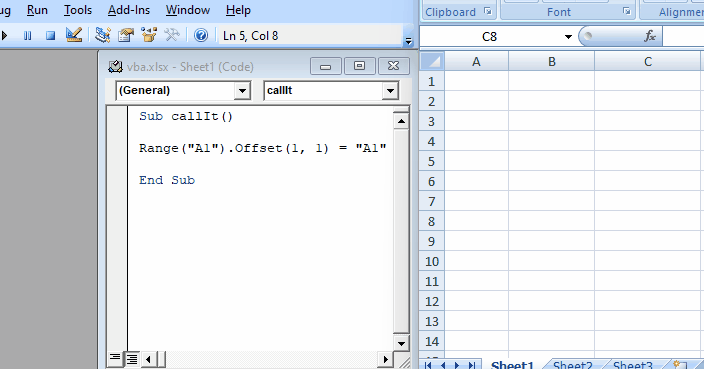Now, try the same scenario using the `Cells` method below.

``````# vba
Sub callIt()

Cells(1, 1).Offset(0, 0) = "A1"

End Sub
``````

Output: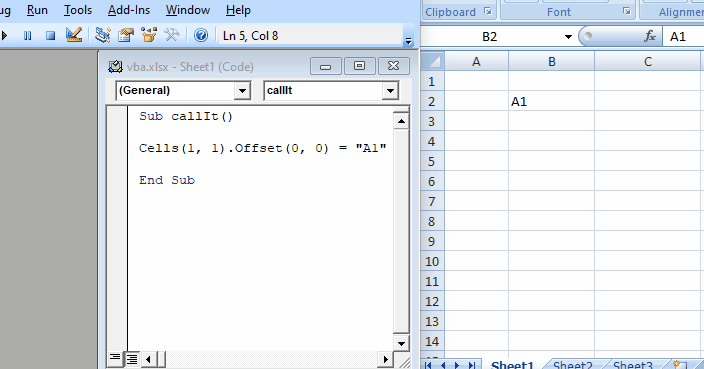Now, let us imagine if we want to add the values to multiple cells based on the offset value of the range, we can do this as shown below.

``````# vba
Sub callIt()

Range("A1:B13").Offset(4, 2) = "Completed"

End Sub
``````

Output: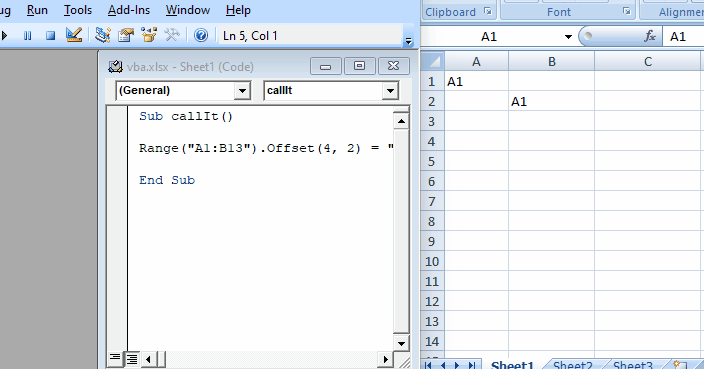## Refer to a Cell Address in VBA

Now, if we want to get the address of a cell, we can quickly get it using the function `Address`. Let’s go through an example in which we will try to get the address of a specific cell, as shown below.

``````# vba
Sub callIt()

Set cell = Cells(2, 4)

End Sub
``````

Output: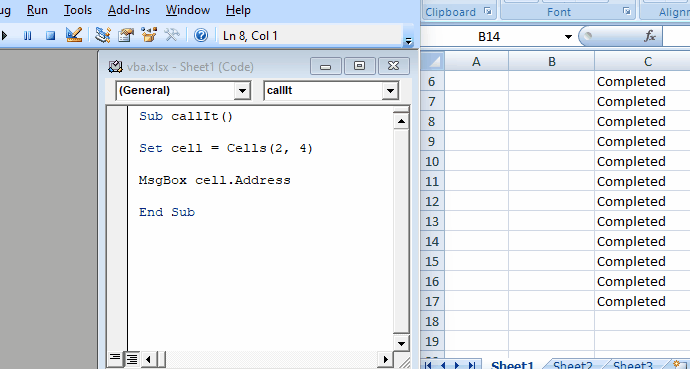As you can see from the above example, we can easily get the address of any cell using the method `address` in VBA.

## Include All Cells in VBA

There may be situations where we want to clear all the data from all cells. For this scenario, we can write the following code as shown below.

``````# vba
Sub callIt()

Cells.Clear

End Sub
``````

Output: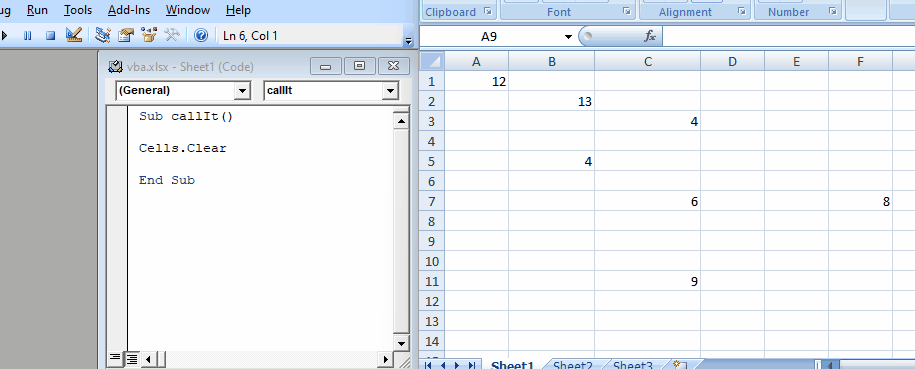As you can see from the above example that we can easily

## Change Properties of a Cell in VBA

There may be situations when we have to change the properties of specific cells. VBA provides easy ways to change the properties of any cell.

Let us go through an example in which we will change the font color, as shown below.

``````# vba
Sub callIt()

Set NewCell = Range("A1")

NewCell.Activate

NewCell.Font.Color = RGB(255, 0, 0)

End Sub
``````

Output: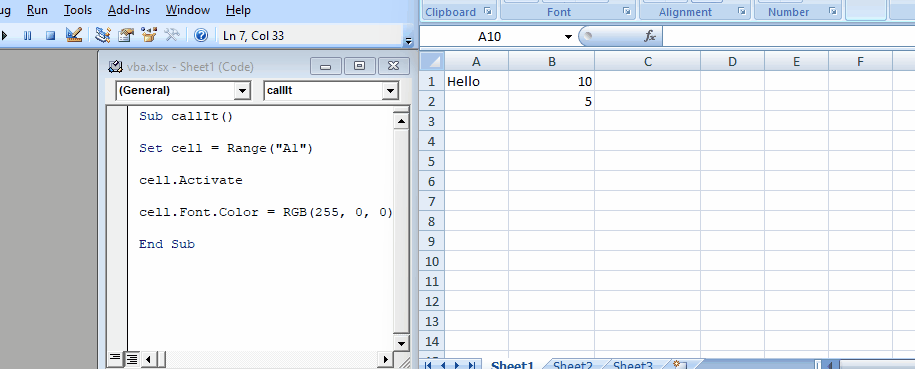Now, let us try to change the font family and font size of the cell, as shown below.

``````# vba
Sub callIt()

Set cell = Range("A1")

cell.Activate

cell.Font.Size = 20
cell.Font.FontStyle = "Courier"

End Sub
``````

Output: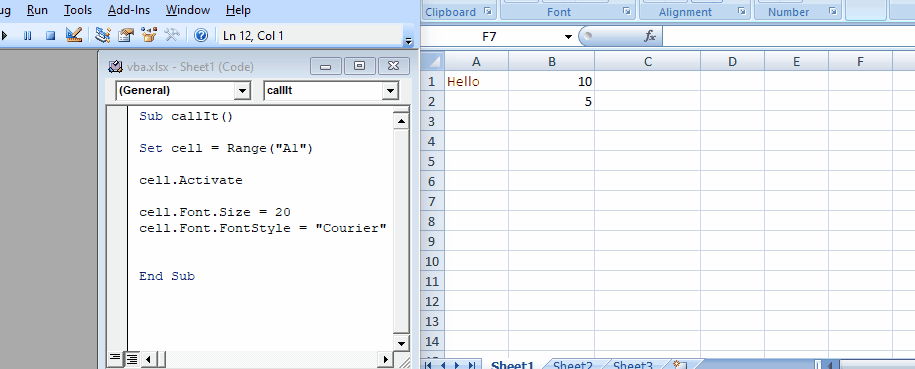## Find the Last Cell in VBA

There may be some situations where we may have to find the last cell of the column or row. We can use `Row.count` and `Column.count` in VBA to find the last row of an excel worksheet.

As shown below, let’s go through an example and find the last cell from rows and columns.

``````# vba
Sub callIt()

MsgBox ("Last cell row wise in the sheet: " & Rows.Count)

MsgBox ("Last cell column wise in the sheet: " & Columns.Count)

End Sub
``````

Output: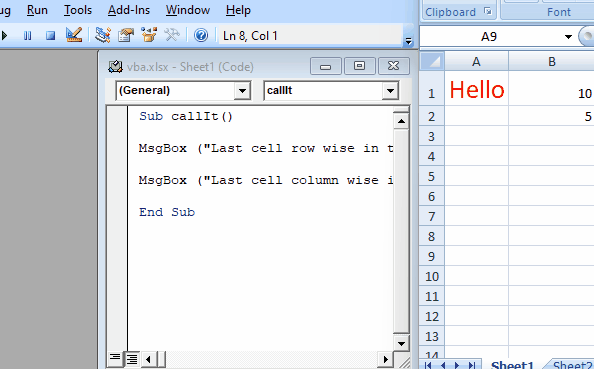As you can see from the above example that it is pretty easy to find the last cell of the row or column by using the simple function `count`.

## Find the Last Cell Used in a Column in VBA

There may be situations when we want to know the last cell used by a column to get an idea of the number of data we are storing.

As shown below, let’s go through an example and find the last cell occupied in specific columns.

``````# vba
Sub callIt()

MsgBox (Cells(Rows.Count, "B").End(xlUp).Row)

End Sub
``````

Output: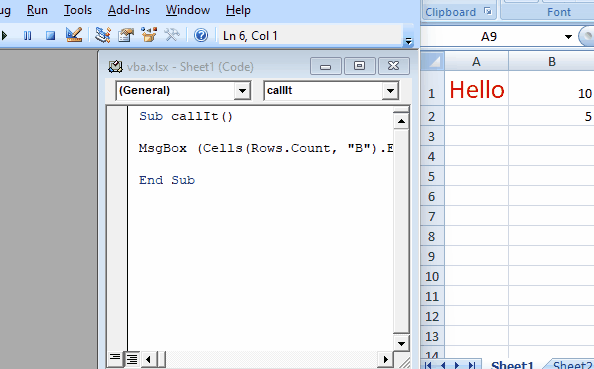As you can see from the above example, we can find the last cell used with 100% accuracy. VBA is the best method to find these things accurately and not lose any data.

## Find the Last Cell Used in a Row in VBA

There may be situations when we want to know the last cell used by a row to get an idea of the number of data we are storing.

As shown below, let us go through an example and find the last cell occupied in a specific row.

``````# vba
Sub callIt()

MsgBox (Cells(9, Columns.Count).End(xlToLeft).Column)

End Sub
``````

Output: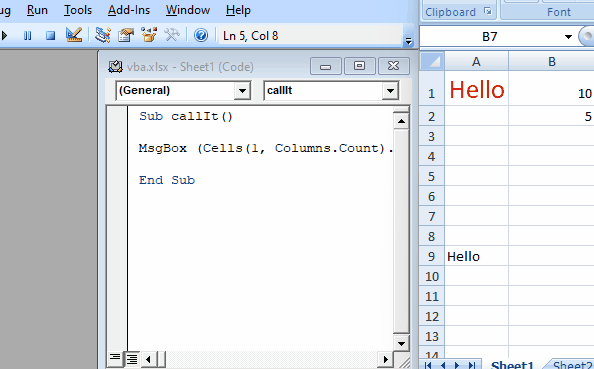As you can see from the above example, we can find the last cell used with 100% accuracy. VBA is the best method to find these things accurately and not lose any data.

## Copy-Paste Cell Values in VBA

There may be situations in which we may have to copy and paste specific values from one cell to another cell. Or we can also copy and paste from one sheet to another sheet with VBA, as shown below.

``````# vba
Sub callIt()

Worksheets("Sheet2").Range("A1:B13").Copy Destination:=Worksheets("Sheet1").Range("A1")

End Sub
``````

Output: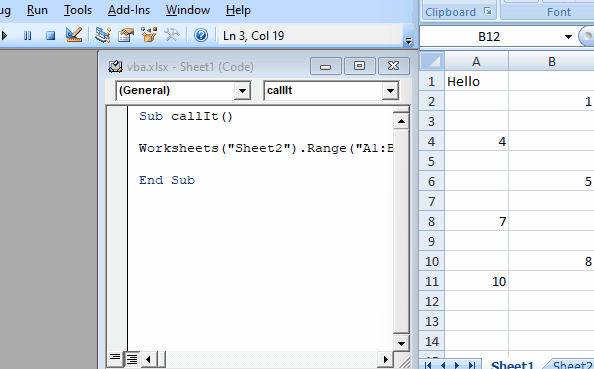As you can see from the above example, we can perform many tasks using cells.

## Related Article - VBA Cell

• Different Ways to Check if a Cell Is Empty in Microsoft Excel VBA
• Change Cell Color in VBA